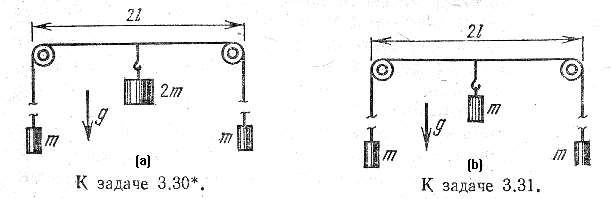### Third Assignment -- Homework problems due Friday, February 10   Solutions here

1. Text, problem 4.36.

2. Use the results of problem 1 to solve the following:
(a) Two equal weights of mass m hang from the ends of a very long string. The string passes over two small massless pulleys that are separated by a distance 2l. A weight of mass 2m is attached to the middle of the string. Find the speed of the weights after a sufficiently long time.*
(b) Similar setup, but the system is set into motion by a central weight of mass m. Find the maximum speed of the central weight, and its maximum distance from its initial position** (which is that shown in the figure).3. Text, problem 1.47 but do it in the following simpler way:
(a) Find an expression for a particle's kinetic energy in cylindrical coordinates.
(b) Substitute into the equations derived last week,
 maq = d dt ćč ¶T ¶q· öř - ¶T ¶q ,
where q = ρ, φ, or z, q· = dq/dt (as usual), and aq is the acceleration component in the (non-unit) basis dq. Convert to the unit vector basis and compare to formula on p. 34, or to the answer in back of book
(If this does not seem simpler, do the problem as the book says.)

4. Text, problem 4.34

5. (a) Suppose you know that the total momentum P of a system of n particles is constant, and also that the total angular momentum L of the system about the origin is constant. Show that then the total angular momentum about any fixed point r = a is also constant.
(b) But the converse is not true: give an example of particle motion (case n=1 is enough) which has constant angular momentum about two different points, but whose linear momentum is not constant.

*In the original:
На концах очень длинной нити подвешены равные грузы масс m. Нить перекинута через два невесомых маленьких блока, расположенных на расстоянии 2l друг от друга. Найдите скорость грузов по истечении достаточно больщого промежутка времени, если к серединие нити приклеплен груз массы 2m.

**Систему, изображенную на рисунке, приводит в движение центральный груз массы m. Определите максимальную скорость центрального груза и максимальное удаление его от начального положения.